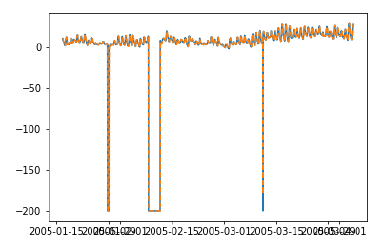# Time Series - Walk Forward Validation

In time series modelling, the predictions over time become less and less accurate and hence it is a more realistic approach to re-train the model with actual data as it gets available for further predictions. Since training of statistical models are not time consuming, walk-forward validation is the most preferred solution to get most accurate results.

Let us apply one step walk forward validation on our data and compare it with the results we got earlier.

In :

prediction = []
data = train.values
for t In test.values:
model = (ExponentialSmoothing(data).fit())
y = model.predict()
prediction.append(y)
data = numpy.append(data, t)


In :

test_ = pandas.DataFrame(test)
test_['predictionswf'] = prediction


In :

plt.plot(test_['T'])
plt.plot(test_.predictionswf, '--')
plt.show()In :

error = sqrt(metrics.mean_squared_error(test.values,prediction))
print ('Test RMSE for Triple Exponential Smoothing with Walk-Forward Validation: ', error)
Test RMSE for Triple Exponential Smoothing with Walk-Forward Validation:  11.787532205759442


We can see that our model performs significantly better now. In fact, the trend is followed so closely that on the plot predictions are overlapping with the actual values. You can try applying walk-forward validation on ARIMA models too.